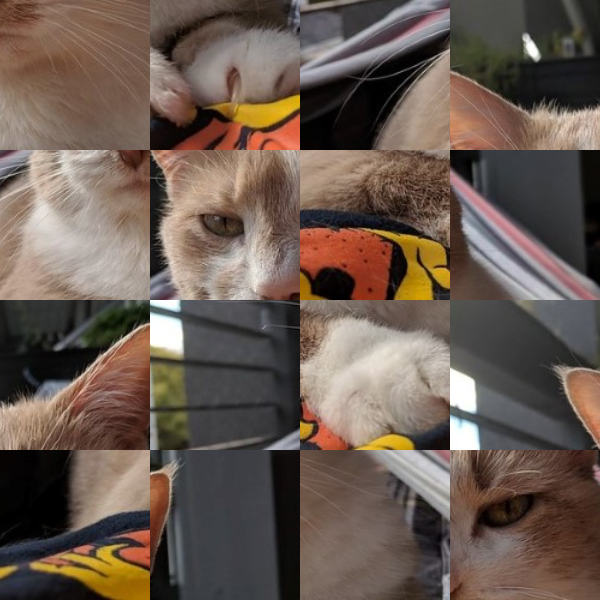# Image Tiles

example p5.js javascript images 🎃

This sketch rearranges an image into a bunch of tiles.

``````let img;

// The squares the image is split into.
let imgSquares = [];

// How many squares should the image be split into?
// Try changing these values!
const horizontalSquareCount = 6;
const verticalSquareCount = 6;

// These variables will hold the size of the image squares.
// We need this to draw the squares in the right places.
let squareWidth;
let squareHeight;

}

function setup() {
createCanvas(600, 600);

// Resize the image so it fits on the screen
img.resize(width, height);

// Calculate the size of the squares.
squareWidth = width / horizontalSquareCount;
squareHeight = height / verticalSquareCount;

// Split the image up into squares.
for (var y = 0; y < height; y += squareHeight) {
for (var x = 0; x < width; x += squareWidth) {
imgSquares.push(img.get(x, y, squareWidth, squareHeight));
}
}

// other setup
pd = pixelDensity();
noLoop();
}

// We called noLoop() above so the draw() function is only called once.
// Click the mouse to redraw the squares in a different order!
function mouseClicked() {
draw();
}

function draw() {

// Randomize the order of the squares
imgSquares = shuffle(imgSquares);

// Keep track of the position of the current square.
// We change these as we draw each square,
// so we know where to draw the next one.
let squareX = 0;
let squareY = 0;
for (const square of imgSquares) {
// Draw this square.
image(square, squareX, squareY);

// Draw the next square to the right of this square.
squareX += squareWidth;
// If the square went off the right edge, move down
// one row and start over at the left edge.
if (squareX >= width) {
squareX = 0;
squareY += squareHeight;
}
}
}
``````This is part of p5 spooky sketches printout I made for CC Fest in 2019. That printout contains a bunch of Halloween-themed examples of drawing and image manipulation. Feel free to use it on your own or in a classroom!

# Remix Ideas

• Rearrange a different image, or change the number of tiles.
• Mix two different images together.
• Make a sliding puzzle!